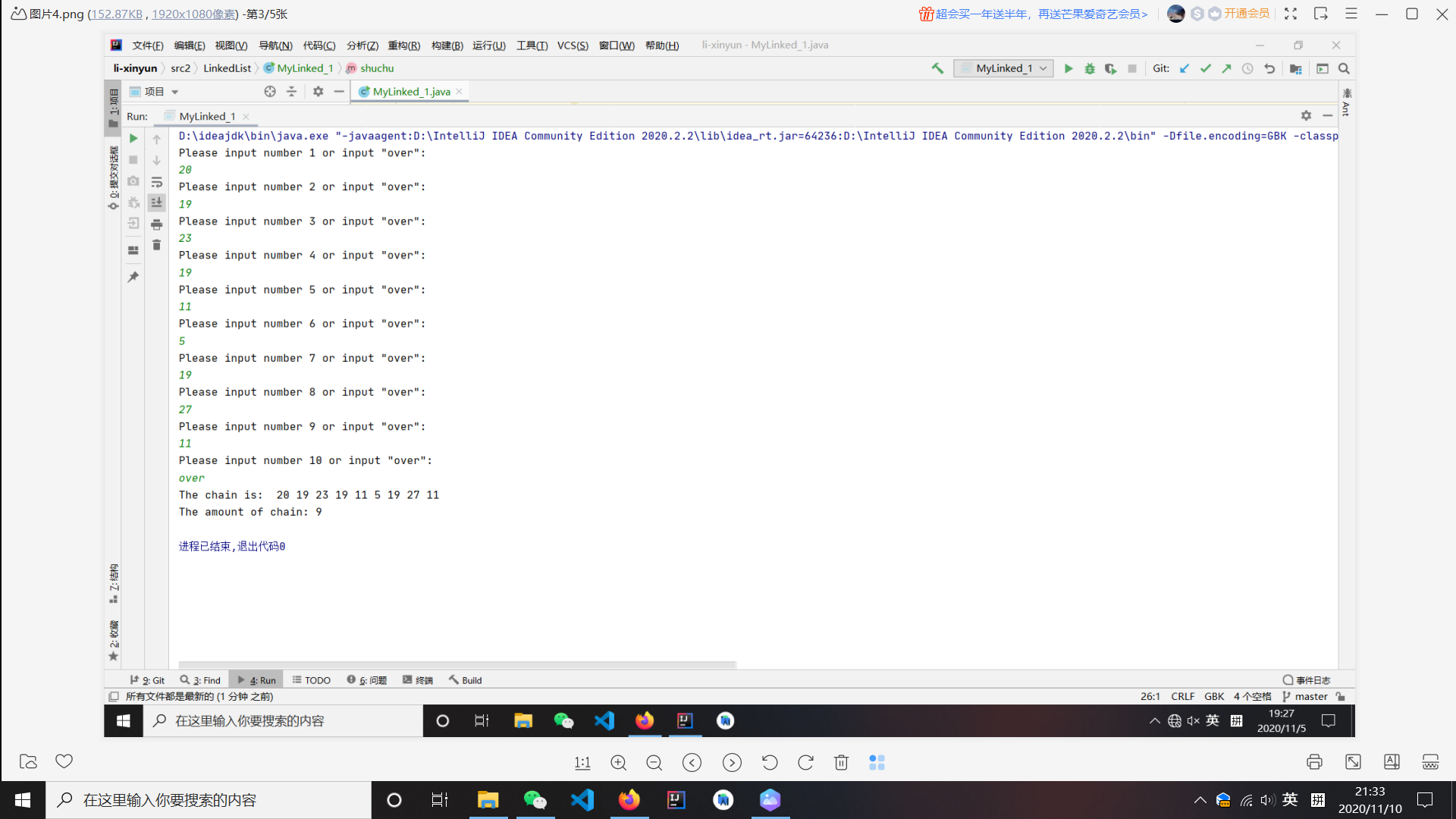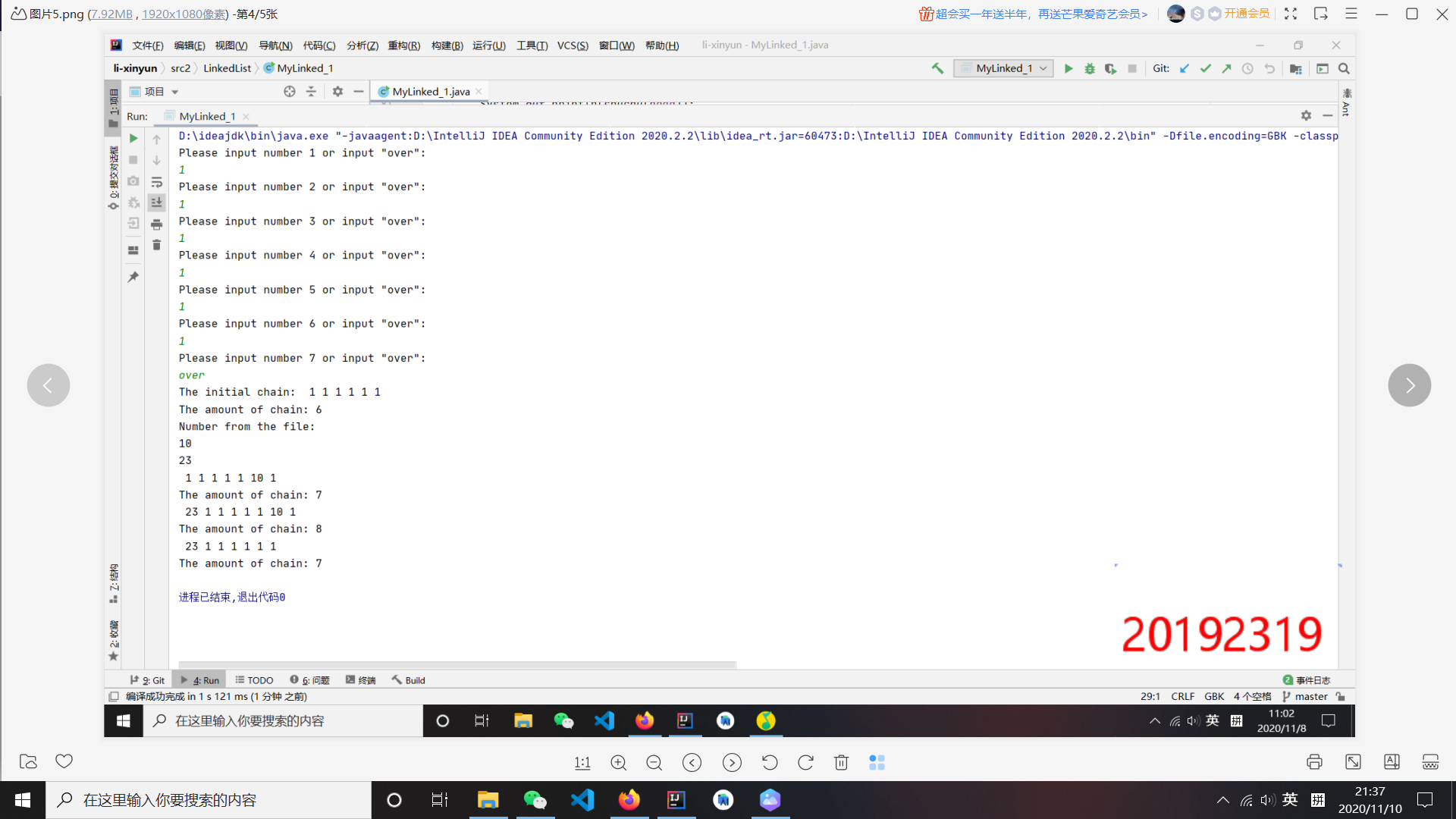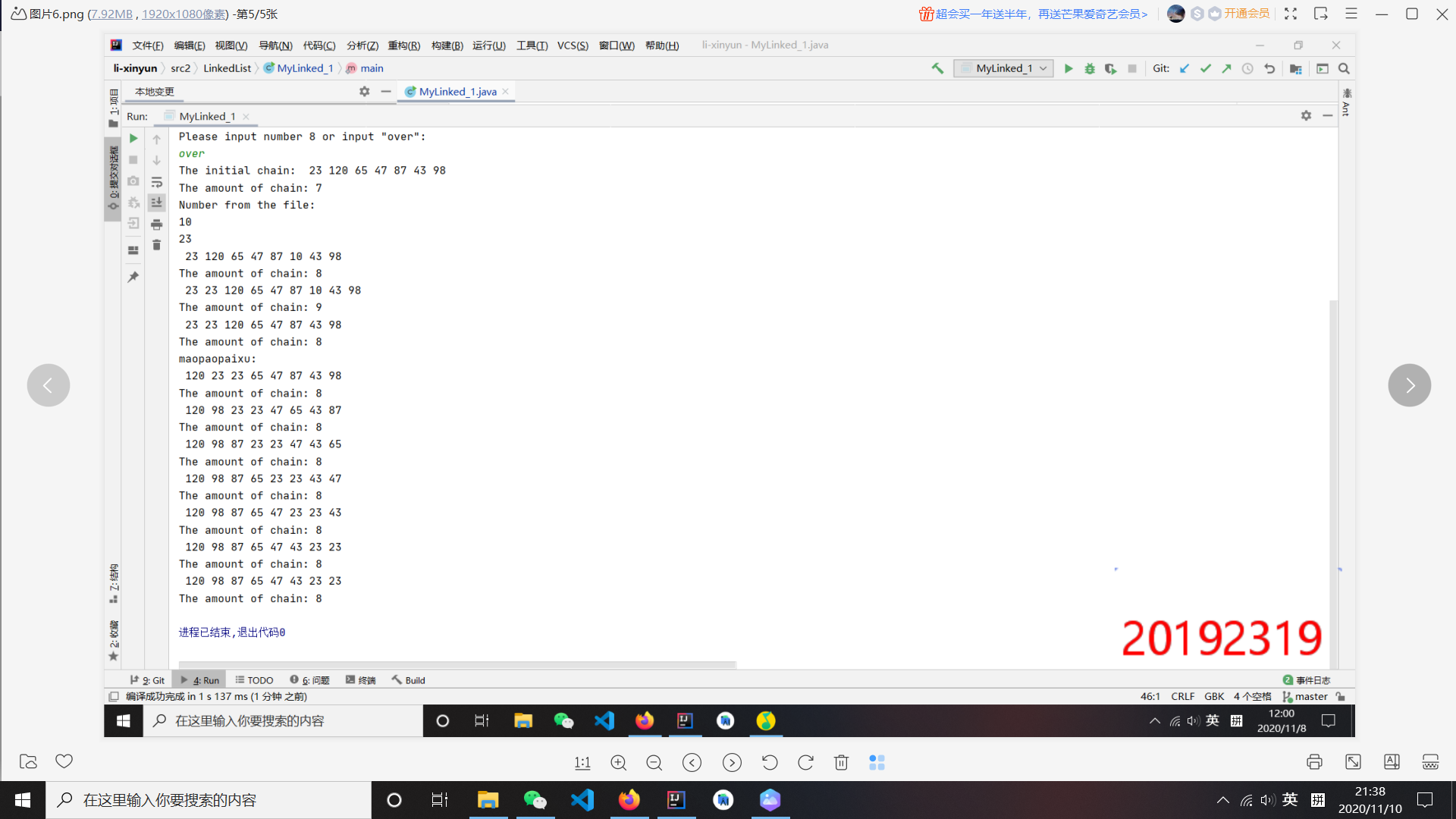# 实验六报告

• 课程：《程序设计与数据结构》
• 班级： 1923
• 姓名： 李歆韵
• 学号：20192319
• 实验教师：王志强
• 实验日期：2020年11月5日
• 必修/选修： 必修

1.链表练习，要求实现下列功能：

2.链表练习，要求实现下列功能：

3.链表练习，要求实现下列功能：

## 2.实验过程与结果

1.链表练习，要求实现下列功能：

package LinkedList;

import java.util.*;
import java.io.*;

public static int nLiXinyun = 0;
public static Chain_1 reserve1;
public static Chain_1 reserve2;

public static void main(String[] args) throws IOException{
Scanner a = new Scanner(System.in);
String b;

System.out.println("Please input number "+((nLiXinyun+1))+" or input \"over\": ");
b = a.next();
while(!b.equals("over")){
nLiXinyun++;
System.out.println("Please input number "+((nLiXinyun+1))+" or input \"over\": ");
b = a.next();
if (b.equals("over")){
break;
}
Chain_1 temp = new Chain_1(Integer.parseInt(b));
}
System.out.println("The amount of chain: "+nLiXinyun);
}2.链表练习，要求实现下列功能：

public static void charu(Chain_1 head, int ele, int point){
Chain_1 node = new Chain_1(ele);
int n =0;
if (point == 0){
}

else {
while (n != point - 1) {
n++;
}
}
nLiXinyun++;
}

public static void shanchu(Chain_1 head, int point){
int n = 0;
if (point == 0){
}
else {
while (n != point - 1){
n++;
}
}
nLiXinyun--;
}3.链表练习，要求实现下列功能：

public static void maopaopaixu(){
Chain_1 c1,c2;
int i,j;
if (temp == null || temp.getNext() == null){
System.out.println("The amount of chain: "+nLiXinyun);
}
for (i=1;i<nLiXinyun;i++){
for (j=i+1;j<=nLiXinyun;j++){

for (int x=1; x<i; x++){
c1 = c1.getNext();
}
for (int y=1; y<j; y++){
c2 = c2.getNext();
}

if (c1.getE1()<c2.getE1()){
int t;
t = c1.getE1();
c1.setE1(c2.getE1());
c2.setE1(t);
}
}
System.out.println("The amount of chain: "+nLiXinyun);
}
}## 参考资料

posted on 2020-11-10 21:45  节奏阿莱士  阅读(78)  评论(0编辑  收藏  举报